8 min read

# animatrixr & Visualizing Matrix Transformations pt. 2

This post is a continuation on my post from last week on Visualizing Matrix Transformations with gganimate. Both posts are largely inspired by Grant Sanderson’s beautiful video series The Essence of Linear Algebra and wanting to continue messing around with Thomas Lin Peterson’s fantastic gganimate package in R.

As with the last post, I’ll describe trying to (very loosely) recreate a small part of the visualizations showing the geometry of matrix multiplication and changing basis vectors (using gganimate in R). (Once again, just in the 2x2 case.)

If you are really interested in building visualizations like the ones shown on 3Blue1Brown, you should check-out the associated manim project on github.

# Topics to cover

I had two major sections in the Appendix of last week’s post:

1. “Multiple matrix transformations”
2. “Potential improvements” (where I mostly describe limitations around visualizing rotations)

This post expands on these topics.

# animatrixr and multiple matrix transformations

Sanderson discusses the value in sometimes decomposing a matrix transformation and thinking of its parts sequentially. I created a toy package animatrixr for building chained matrix transformations that can then be animated using gganimate1.

The function animatrixr::add_transformation() lets you chain together matrix transformations with R’s pipe operator %>%.

For example, let’s consider three matrix transformations: horizontal sheer –> vertical sheer –> reflection across x-axis:

library(dplyr)

if (!requireNamespace("animatrixr")) devtools::install_github('brshallo/animatrixr')
library(animatrixr)
sheer_horizontal <- tribble(~ x, ~ y,
1, 0.5,
0, 1) %>%
as.matrix()

sheer_vertical <- tribble(~ x, ~ y,
1, 0,
0.5, 1) %>%
as.matrix()

reflect_x <- tribble(~ x, ~ y,
1, 0,
0, -1) %>%
as.matrix() 

Now let’s visualize the transformations being applied sequentially:

matrix(c(1,0,0,1), nrow = 2) %>%
add_transformation(sheer_horizontal) %>%
add_transformation(sheer_vertical) %>%
add_transformation(reflect_x,
seq_fun = animatrixr::seq_matrix_l,
n_frames = 40) %>%
animate_matrix(datasaurus = TRUE)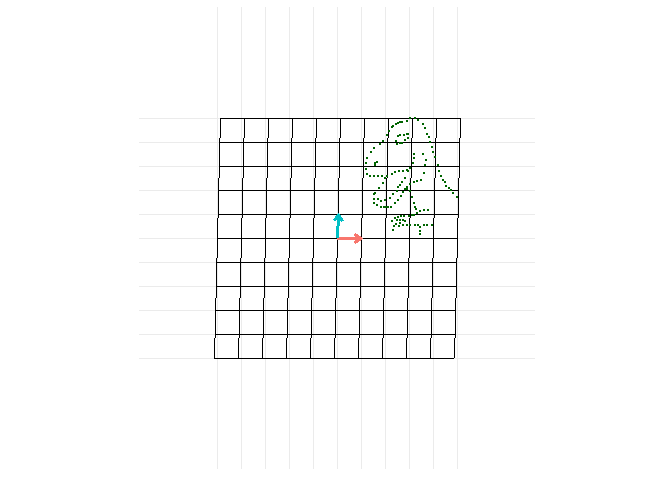add_transformation() explicitly creates in-between frames for a given transformation. The seq_fun argument allows you to define the interpolation method, for example whether the coordinates should (during the animation) follow a linear path (default) or the angle of a rotation.

It would be nice to add-in functionality where the final transformation object could then be added to layers of a ggplot (though I’ve done nothing towards this except add an argument in animatrixr::animate_matrix() for displaying the datasauRus).

(Warning: animatrixr is severely limited, as discussed in the Appendix and in package documentation. However you can find it at the “brshallo/animatrixr” repo on my github page.)

# Visualizing rotations

The seq_fun argument within add_transformation() specifies frames in-between the start and end states after a matrix transformation. By default it uses animatrixr::seq_matrix_l which changes in-between coordinates linearly (as does gganimate2).

Let’s look at a rotation where the in-between coordinates are interpolated linearly:

rotate_90 <- tribble(~ x, ~ y,
cos(pi / 2), -sin(pi / 2),
sin(pi / 2), cos(pi / 2)) %>%
as.matrix()

matrix(c(1,0,0,1), nrow = 2) %>%
add_transformation(rotate_90) %>%
animate_matrix(datasaurus = TRUE)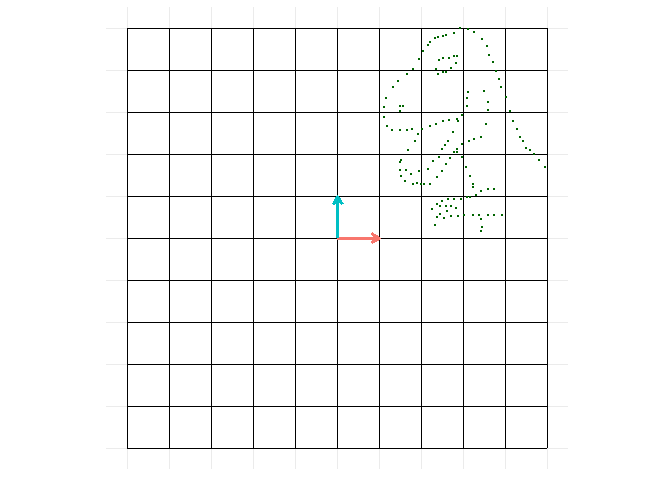Linear interpolation makes the rotation transformation appear scrunched during the animation (from how we intuitively think of a rotation) as the coordinate points take a straight line path to their positions after applying the transformation3.

To make the in-between coordinates instead follow the angle of rotation we could change the seq_fun from animatrixr::seq_matrix_l to animatrixr::seq_matrix_lp.

matrix(c(1,0,0,1), nrow = 2) %>%
add_transformation(rotate_90, seq_fun = animatrixr::seq_matrix_lp) %>%
animate_matrix(datasaurus = TRUE)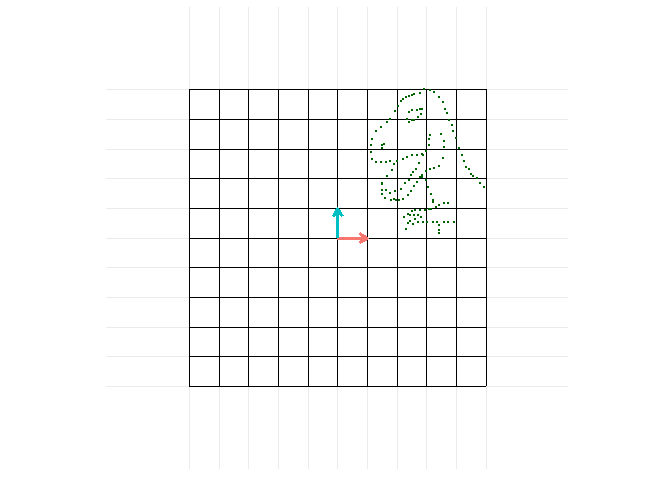During the rotation portion of the animation gganimate is still tweening images linearly, however the frames add_transformation() creates are now following along the angle of rotation of the transformation. Hence the animation ends-up approximating a curved path.

However, seq_matrix_lp() needs improvement and was just set-up to work for toy examples – it really only looks ‘right’ if doing rotations off of $\left(\begin{array}{cc} 1 & 0\\0 & 1 \end{array}\right)$ See Showing rotations in the Appendix for additional detail on how this is set-up and the various limitations with animatrixr.

Happy animatrixing!

# animatrixr::rotation_matrix() is helper function for creating matrix
# transformations of rotations
matrix(c(1,0,0,1), nrow = 2) %>%
add_transformation(animatrixr::rotation_matrix(pi / 2),
seq_fun = animatrixr::seq_matrix_lp) %>%
add_transformation(matrix(c(1, 0.5, 0, 1), nrow = 2)) %>%
add_transformation(matrix(c(1, 0, 0, -1), nrow = 2)) %>%
animate_matrix(datasaurus = TRUE)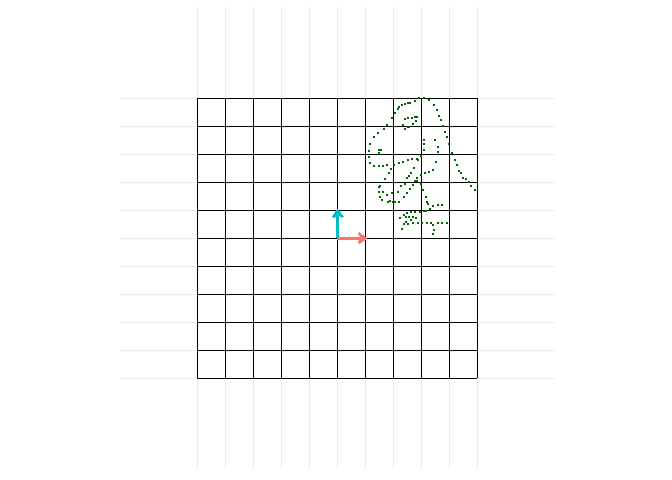# Appendix

## Using animatrixr?

This is a toy package (very hastily written). I have not put effort into thinking about making it usable for others. Also, some parts just don’t really work or aren’t set-up quite right… (as noted in the README and elsewhere in the package). But feel free to check-it out / improve it / make something better! Let me know if you do!

This has been a fun dabble into thinking (at least surface level) about animation. Though I don’t have any plans to add onto this (or write any more posts on this topic). If I do add anything, it will most likely just be cleaning-up the decomposition methods in the seq_matrix*() functions. But no plans4

## Notes on seq functions

Below are additional notes on the animatrixr::seq_matrix* functions. They need some work, but here is a description of how they are currently set-up.

### Showing rotations

To animate the rotation of a transformation, add_transformation(m = matrix(c(0, 1, -1, 0), nrow = 2), seq_fun = seq_matrix_lp) explicitly creates in-between frames on the path the points would follow if they were instead following polar coordinates along the angle of rotation. In the next few sections I’ll discuss the process for doing this (again, this is not necessarily an ideal set-up).

Given any 2x2 matrix:

$\left(\begin{array}{cc} a & b\\ c & d \end{array}\right)$

you can use the equation atan2(c, a) to extract the angle of rotation from the matrix5 and then create a sequence from the starting angle of rotation to the final angle of rotation.

For example, if my start angle is $$0^\circ$$, and final angle of rotation is at $$38^\circ$$ and I have 20 frames, then my sequence would be:

$0^\circ, 2^\circ, ... 38^\circ$

A rotation matrix is defined as:

$\left(\begin{array}{cc} cos(\theta) & -sin(\theta)\\ sin(\theta) & cos(\theta) \end{array}\right)$

Hence I can convert my sequence of angles into a sequence of matrices that define the rotations applied for each explicit in-between frame.

$\left(\begin{array}{cc} cos(0^\circ) & -sin(0^\circ)\\ sin(0^\circ) & cos(0^\circ) \end{array}\right), \left(\begin{array}{cc} cos(2^\circ) & -sin(2^\circ)\\ sin(2^\circ) & cos(2^\circ) \end{array}\right)... \left(\begin{array}{cc} cos(28^\circ) & -sin(28^\circ)\\ sin(28^\circ) & cos(28^\circ) \end{array}\right)$

### seq_matrix_lp applied on non-standard unit basis vectors

If you input a matrix transformation into seq_matrix_lp that is not a pure rotation from the unit vectors it will decompose the matrix into a rotation component and other component6, the other component creates a sequence of matrices that have the in-between frames interpolated linearly. The sequence of rotation and other matrices are then recomposed to provide the final sequence.

This approach means that non-pure rotations on the unit vectors, etc. will not really look like rotations. I would need to factor in other components (e.g. scale) to improve this.

### Show rotation first

Beyond seq_matrip_l() and seq_matrix_lp(), I made another seq_matrix* function: seq_matrix_rotate_first which (like seq_matrix_lp) also decomposes a matrix into rotation and other components. Rather than interpolating these separately and then recomposing them (as seq_matrix_lp does) seq_matrix_rotate_first works by interpolating them separately and then applying the decomposed sequences sequentially – so the entire rotation component of the transformation will be animated and then the ‘other’ component will be animated (this makes for twice as many frames when there is a ‘rotation’ and ‘other’ component in the transformation matrix).

I.e. starting from our identity matrix and applying a single matrix transformation, it will automatically decompose this and animate the decomposed parts in two steps, $$I$$ –> $$R$$ and then from $$R$$ –> $$M$$. Below is an example of the animation for the transformation matrix: $\left(\begin{array}{cc} 0 & -1\\1 & -0.5 \end{array}\right)$ (which could be decomposed into a rotation and a sheer part).

transformation_matrix <- sheer_vertical %*% animatrixr::rotation_matrix(pi/4)

matrix(c(1,0,0,1), nrow = 2) %>%
add_transformation(transformation_matrix, seq_fun = seq_matrix_rotate_first) %>%
animate_matrix(datasaurus = TRUE)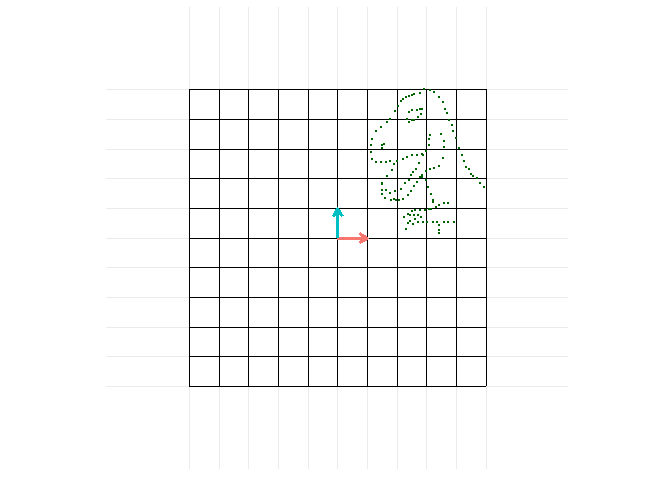There are (especially) a lot of problems with this function currently and I don’t recommend using it e.g.

• only works (at all correctly) if starting from standard unit vectors (hence cannot really be combined into a chain of matrix transformations)
• rotation component extracted will vary depending on what ‘other’ is within M E.g. if M = {rotation}{vertical sheer} vs. M = {rotation}{horizontal sheer} – rotation component will look different
• I defaulted the amount of frames given to the rotation component to be the same as the amount of frames given to other component. If the size of the rotation is small relative to the other part of the transformation (or vice versa) the timing will feel slow/jumpy.

1. Provides a cleaner approach for doing this compared to the clunky method I walked through in my post last week.

2. All visualizations from last week used this linear interpolation method.

3. I discuss this at more length in my previous post – see the sub-section in the “Appendix”, “Problem of squeezing during rotation”.

4. However I also hadn’t planned on writing a follow-up post… so who knows…

5. See post referencing this.

6. To find the ‘other’ component of a matrix transformation… say M represents the overall matrix transformation, in Showing rotations I described how to calculate R (the rotation component), hence to calculate A, ‘other’, I do:

$AR = M$ $ARR^{-1} = MR^{-1}$ $A = MR^{-1}$